Function Repository Resource:

# PermutationSkewSum

Compute the skew sum of permutation lists

Contributed by: Motoharu Ito
 ResourceFunction["PermutationSkewSum"][perm1,perm2,…] gives the skew sum of the permutations permi.

## Details

The skew sum of two permutations p=p1,p2,,pm and q=q1,q2,,qn is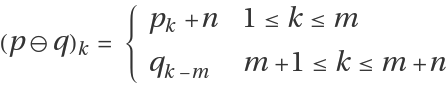.

## Examples

### Basic Examples (2)

The skew sum of {1,4,3,2,5} and {4,2,3,1}:

 In:=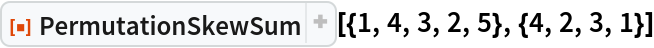Out=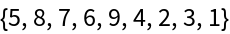The skew sum of four permutations:

 In:=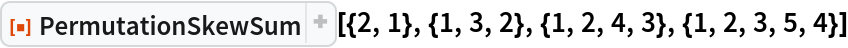Out=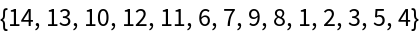### Properties and Relations (2)

The skew sum is not commutative:

 In:=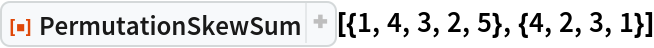Out=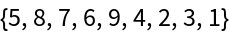In:=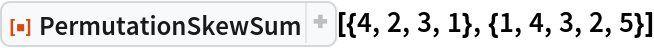Out=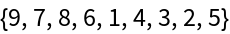The skew sum is associative:

 In:=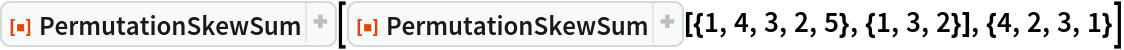Out=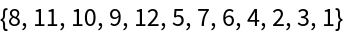In:=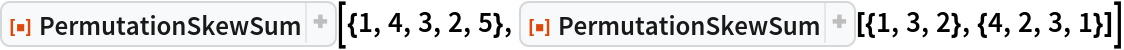Out=Motoharu Ito

## Version History

• 1.0.0 – 29 November 2022